# Analyzing Distances Between Two Sets of ElementsThis task shows how to analyze the distance between any two geometric elements, or between two sets of elements.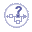The different mapping analyses of the same surfaces cannot be displayed simultaneously, even if you have set the mapping analyzes in no show. You need to visualize them one after the other.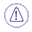This command is only available with: FreeStyle Shaper Wireframe and Surface for Building 1.When computing the distance between two curves, there is no negative values possible as opposed to when analyzing the distance between a surface and another element. Indeed, surfaces present an orientation in all three space directions whereas, in the case of planar curves for example, only two directions are defined. Therefore the distance is always expressed with a positive value when analyzing the distance between two curves. The element which dimension is the smallest (0 for points,1 for curves, 2 for surfaces for example) is automatically discretized, if needed. When selecting a set of element, the system compares the greatest dimension of all elements in each set, and discretizes the one with the smallest dimension.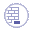Open the FreeStyle_Part_11.CATPart document.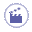Select Curve.1. Click the Distance Analysis icon: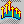The Distance dialog box appears, the Second set state is selected: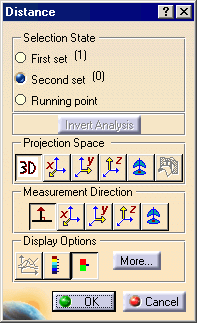The Distance dialog box displays the following options: Selection State: defines the elements to be analyzed. To add or remove an element in a set, use the Ctrl key. First set: defines the first set of elements to be analyzed. All the elements selected before running the command are considered in the First set, the Second set is then automatically selected. Second set: defines the second set of elements to be analyzed. Running point: allows you when you move the pointer over the discretized element to display more precise distance value between the point below the pointer and the other set of elements.  The projection is visualized and the value is displayed in the geometry area. Note that the analyzed point is not necessarily a discretized point in this case. This is obvious when a low discretization value is set, as shown here. Invert Analysis: inverts the computation direction. In some cases, when inverting the computation direction does not make sense, when one of the elements is a plane for example, the Invert Analysis button is grayed. Projection Space: defines the preprocessing of the input elements used for the computation.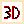3D: elements are not modified and the computation is done between the initial elements.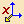X: projection according to the X axis, the computation is done between the projection of selected elements. Only available when analyzing distances between curves.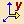Y: projection according to the Y axis, the computation is done between the projection of selected elements. Only available when analyzing distances between curves.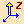Z: projection according to the Z axis, the computation is done between the projection of selected elements. Only available when analyzing distances between curves.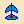Compass: projection according to the compass current orientation, the computation is done between the projection of selected elements.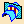Planar distance: the distance is computed between a curve and the intersection of the plane containing that curve. Only available when analyzing distances between a curve and a plane. Measurement Direction: provides options to define how set the direction used for the distance computation.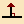Normal distance : the distance is computed according to the normal to the other set of elements.X: the distance is computed according to the X axis.Y: the distance is computed according to the Y axis.Z: the distance is computed according to the Z axisCompass: the distance is computed according to the compass orientation. Display Options: defines the display options.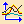2D Diagram: displays a 2D diagram representing the distance variation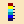Full color range: makes a complete analysis based on the chosen color range. This allows you to see exactly how the evolution of the distance is performed on the selected element, this functionality is P2-only.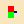Limited color range: makes a simplified analysis, with only three values and four colors, display by default. Click the More button in the Distance dialog box.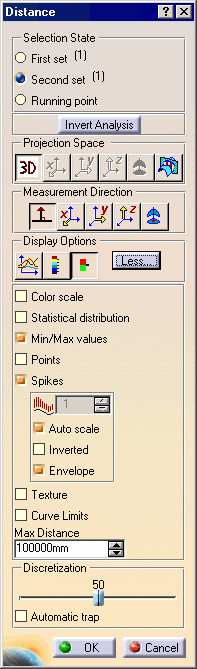The Distance dialog box displays the following extended options: Color scale: to display the Distance.x dialog box whether the full or the limited color range. Statistical distribution: displays the percentage of points between two values, effective when the Color scale option is selected. Min/Max values: displays the minimum and maximum distance values and locations on the geometry. Points: displays the distance analysis in the shape of points only on the geometry. Spikes: displays the distance analysis in the shape of spikes on the geometry. You can further choose to: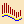sets the ratio for the spike size, available only when the Auto scale option is unselected. Auto scale: sets an automatic optimized spike size. Inverted: inverts the spike visualization on the geometry Envelope: displays the envelope, that is the curve connecting all spikes together Texture: checks the analysis using color distribution: This option is only available with surface elements in at least one set, providing this set is discretized. The distance is computed from this discretized set to the other set. The texture mapping is computed on the discretized surface. It is not advised to use it with planar surfaces or ruled surfaces. A user defined color without associated value is added in the full or the limited color range, which allows you to manage an independent color for no valuated area. Statistical distribution, Min/Max values, and Points cannot be visualized when using the Texture option. The discretization option should be set to a maximum (in Infrastructure User's Guide, see Improving Performances, the 3D Accuracy > Fixed option should be set to 0.01). Run the View > Render Style > Customize View command, and select in the Custom View Modes dialog box which appears the following options to be able to see the analysis results on the selected element. Otherwise a warning is issued: Select the Edges and points option then the All edges option. Select the Shading option then the Material option. Curve Limits: re-limits the discretized curve, this functionality is P2-only. Max Distance: re-limits the maximal distance value. Discretization: reduces or increases the number of points of discretization from the set of elements which are taken into account when computing the distance. Automatic trap: delimits the set of points of discretization to be taken into account for the computation, in the case of a large set of points of discretization, thus improving the performances. When using the Automatic trap option, a mean plane representing the delimited set of points of discretization is computed from their distribution. If this distribution is too scattered, this option may not be able to take into account as many points as needed for a consistent result such as an incomplete result or no result. In this case, it is best to unselect the option. Select Surface.1. Select Running Point and move the pointer over the discretized element.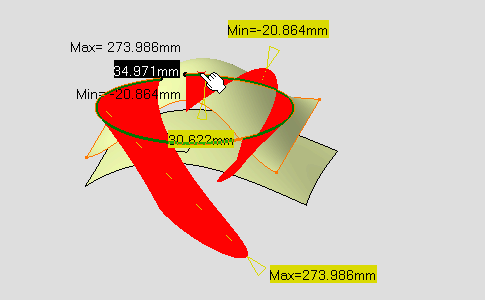Select the Color Scale option. The Distance.1 dialog box appears and shows the Limited color range and identifies the maximum and minimum values for the analysis.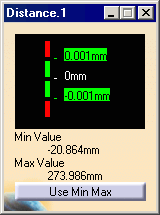The distance analysis is computed. Each color identifies all discretization points located at a distance between two values, as defined in the Limited color range dialog box.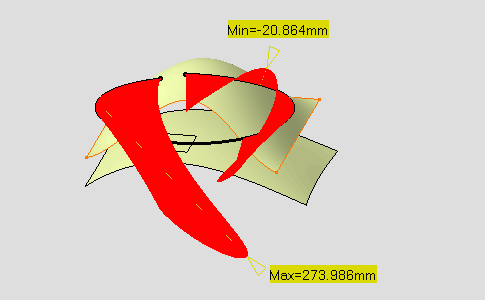Select the Full color range option:The Distance.1 dialog box shows the Full color range and identifies the maximum and minimum values for the analysis.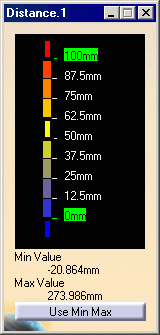The distance analysis is computed. Each color identifies all discretization points located at a distance between two values, as defined in the Full color range dialog box.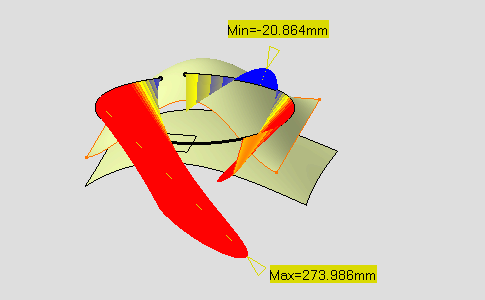The following description is valid for the Limited color range and the Full color range dialog boxes. Whichever mode you choose the use of the color scale is identical: it lets you define colors in relation to distance values. You can define each of the values and color blocks, therefore attributing a color to all elements which distance falls into to given values. You can right-click on a color rectangle in the color scale to display the contextual menu: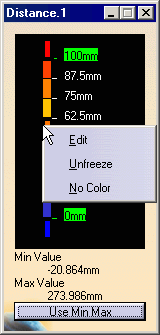Edit contextual command allows you to modify the values in the color range to highlight specific areas of the selected surface. The Color dialog box is displayed allowing the user to modify the color range.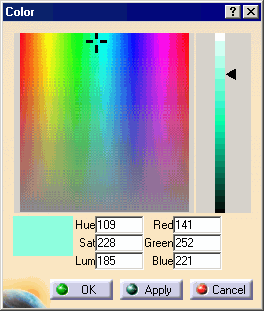Unfreeze contextual command allows you to perform a linear interpolation between non defined colors.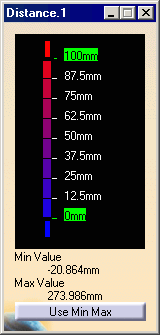No Color contextual command can be used to simplify the analysis, because it limits the number of displayed colors in the color scale. In this case, the selected color is hidden, and the section of the analysis on which that color was applied takes on the neighboring color.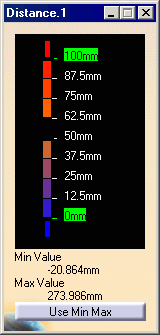You can also right-click on the value to display the contextual menu: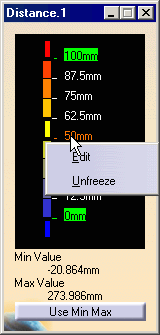Edit contextual command allows you to modify the edition values. The Value Edition dialog box is displayed: enter a new value (negative values are allowed) to redefine the color scale, or use the slider to position the distance value within the allowed range, and click OK. The value is then frozen, and displayed in a green rectangle.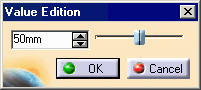Unfreeze contextual command allows you to perform a linear interpolation between non defined values, meaning that between two set (or frozen) colors/values, the distribution is done progressively and evenly. This command is available for all values except for maximum and minimum values. The unfreezed values are no longer highlighted in green. Use Max / Use Min contextual commands allow you to evenly distribute the color/value interpolation between the current limit values, on the top/bottom values respectively, rather than keeping it within default values that may not correspond to the scale of the geometry being analyzed. Therefore, these limit values are set at a given time, and when the geometry is modified after setting them, these limit values are not dynamically updated. These commands are available for maximum and minimum values only. The Use Max command is available if the maximum value is higher or equal to the medium value, otherwise you need to unfreeze the medium value first. The Use Min command is available if the minimum value is lower or equal to the medium value, otherwise you need to unfreeze the medium value first. Use Min Max button in the Distance Analysis.1 dialog box makes in one action the Use Max / Use Min contextual commands operation. The Distance Analysis.1 is created in the specification tree under the Free Form Analysis.1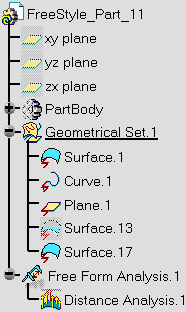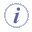The color scale settings (colors and values) are saved when exiting the command, meaning the same values will be set next time you edit a given distance analysis capability. However, new settings are available with each new distance analysis. Click the Use Min Max button in the Distance.1 dialog box. Maximum and minimum values are set according to the computed values displayed below the color scale.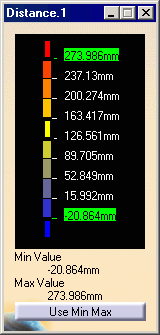The color range has been redefined according to the color scale.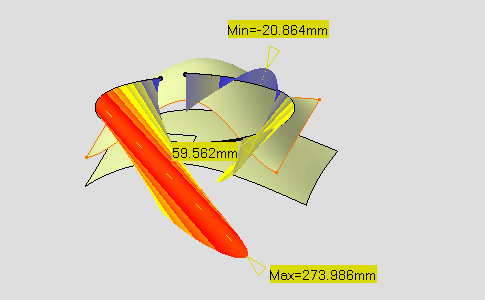Select the Planar distance option:Distance is computed between Curve.1 and the intersection of the plane containing that curve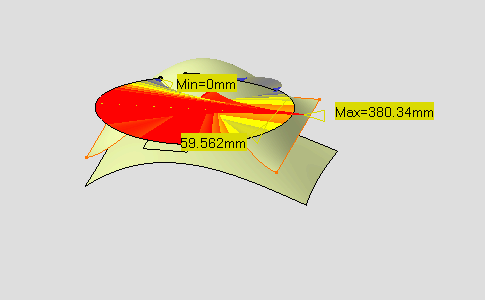Select the 3D option:and the X option in the Measurement Direction:Distance is computed along the X direction.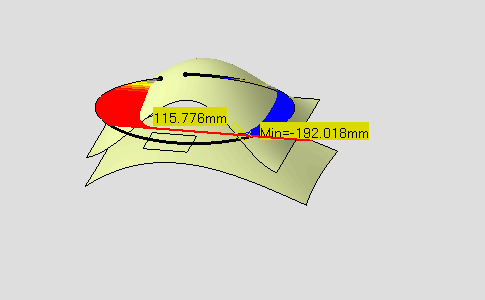Select the Normal Direction option:and the 2D Diagram option:The Distance Analysis.1 dialog box appears and allows you to visualize the distance evolution.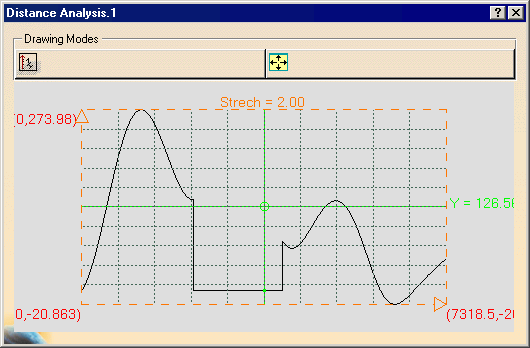The Distance Analysis.1 dialog box displays the following options: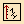Vertical Inverse Scale: draws the curves in a linear horizontal scale and and inverse vertical scale.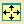Reframe: reframes the frame. Select the Limited color range option:and select the Statistical distribution option.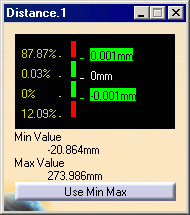Select the Points option and unselect the Spikes option. Show the distance analysis in the shape of points only on the geometry.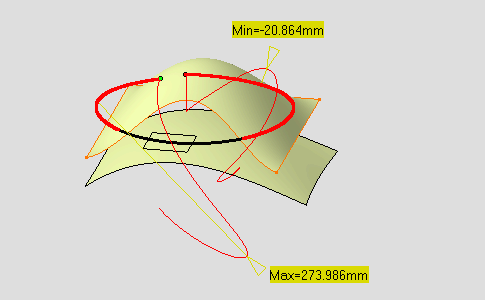Select the Curve Limits option. Two manipulators appear at both extremities of the curve: they let you define new start and end points on the curve.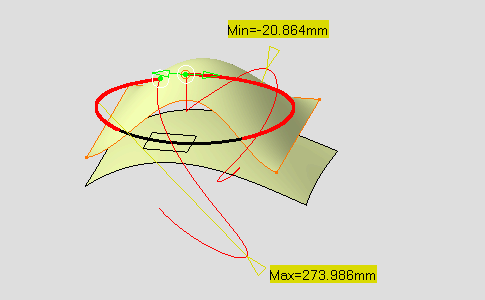Start and end points are defined by a ratio of curve length between 0 and 1. If you extend the curve for instance, this ratio is kept.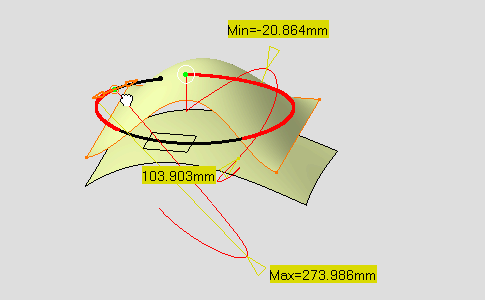Set the Max Distance value to 220mm. The maximum value is re-limited accordingly on the geometry.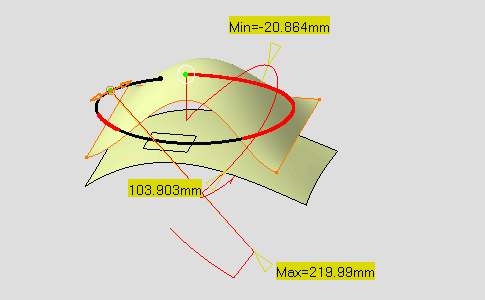Select the First set state and unselect the Curve.1 using the Ctrl key, then select Surface.17. Select the Texture option, the Spikes option is automatically deselected. Select the Full color range option:and click the Use Min Max button in the Distance.1 dialog box. The user defined color is displayed in the Distance.1 dialog box.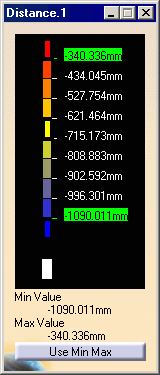The texture mapping is displayed on Surface.17.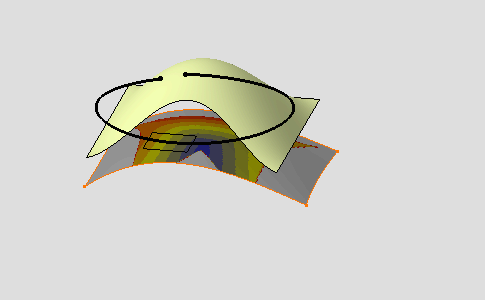Define the user color as magenta in the Distance.1 dialog box.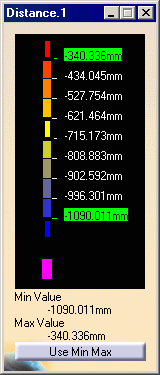The non valuated area is magenta-colored.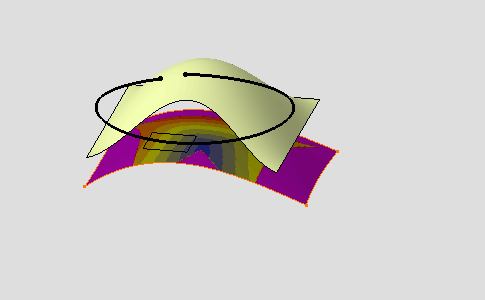Click OK to exit the analysis while retaining it. The analysis (identified as Distance Analysis.x) is added to the specification tree.Even though you exit the analysis, the color scale is retained till you explicitly close is. This is like a shortcut allowing you to modify one of the analyzed elements, which leads to a dynamic update of the distance analysis, while viewing the set values/colors at all times and without having to edit the distance analysis. When analyzing clouds of points, in normal projection type, the distances are computed as the normal projection of each point of the first cloud onto the triangle made by the three points closest to that projection onto the second cloud. As it is a projection, using the Invert Analysis button does not necessarily gives symmetrical results. When you select the geometrical set as an input in the specification tree, all the elements included in this geometrical set are automatically selected too.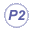The auto detection capability is available from the Dashboard. You can calculate the minimum distance between two curves along a direction using the Knowledge Expert product. For further information, refer to the Knowledge Expert's User's Guide, Reference, Functions Package, Measures chapter.